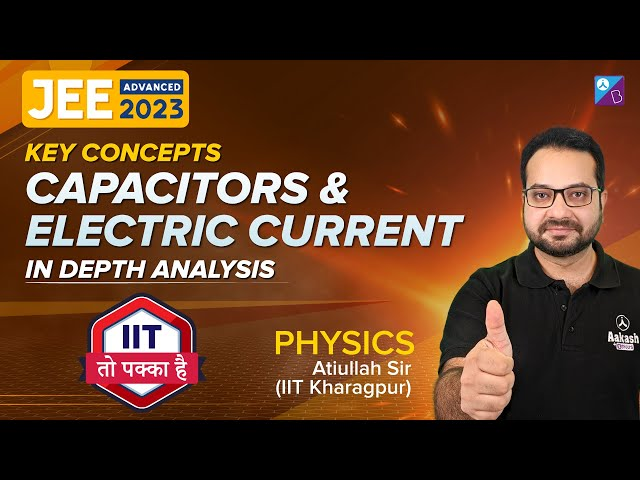Win up to 100% scholarship on Aakash BYJU'S JEE/NEET courses with ABNAT Win up to 100% scholarship on Aakash BYJU'S JEE/NEET courses with ABNAT

# JEE Advanced Previous Year Questions with Solutions on Capacitor

A capacitor is an electrical device for storing a large quantity of charge, and hence electrical energy. A capacitor in its simplest form consists of two parallel conductors of any arbitrary shape separated by a small distance with a dielectric medium in between. The capacitance of the capacitor is defined as the ratio of the magnitude of the charge on either conductor to the potential difference between the conductors forming the capacitor.

Question 1) In the circuit shown below, the switch S is connected to position P for a long time so that the charge on the capacitor becomes q1 µC. Then S is switched to position Q. After a long time, the charge on the capacitor is q2 µC.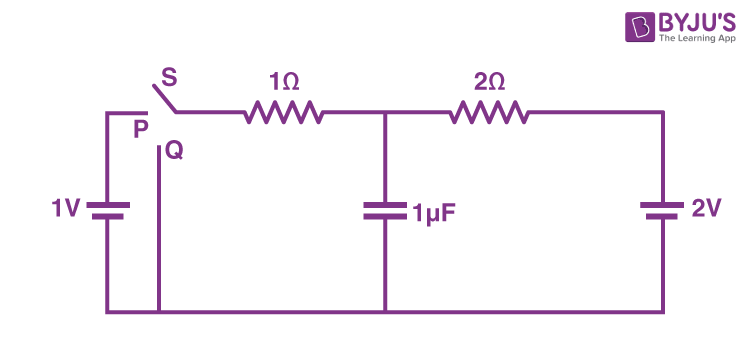The magnitude of q1 is

.

Solution:

With switch S at position P after long time potential difference across capacitor branch

$$\begin{array}{l}\frac{\frac{2}{2}+\frac{1}{1}}{\frac{1}{2}+\frac{1}{1}}=\frac{2\times 1}{3}=(4/3) v\end{array}$$

Charge on capacitor q1μC = (4/3) μC

⇒q1 = 4/3 = 1.33

Question 2) A long straight wire carries a current, l = 2 ampere. A semi-circular conducting rod is placed beside it on two conducting parallel rails of negligible resistance. Both the rails are parallel to the wire. The wire, the rod and the rails lie in the same horizontal plane, as shown in the figure. Two ends of the semicircular rod are at distances 1 cm and 4 cm from the wire. At time t = 0, the rod starts moving on the rails with a speed v = 3.0 m/s (see the figure).

A resistor R = 1.4 and a capacitor C0 = 5.0 F are connected in series between the rails. At time t = 0, C0 is uncharged. Which of the following statement(s) is(are) correct? [μ0 = 4 × 10–7 SI units. Take ln 2 = 0.7]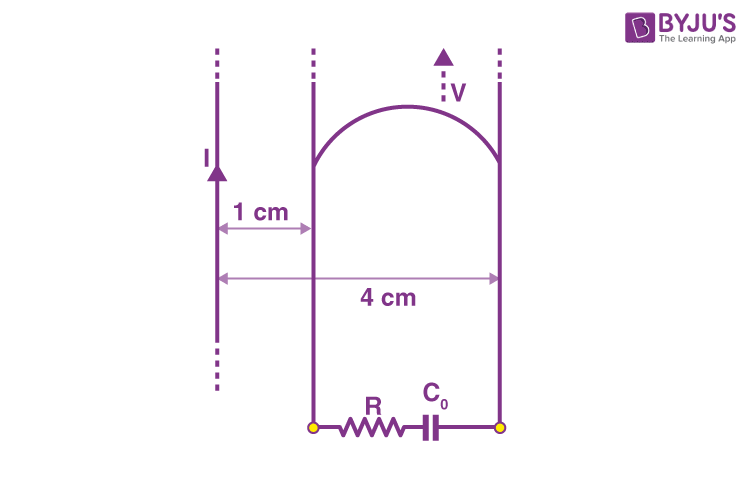(A) Maximum current through R is 1.2 × 10–6 ampere

(B) Maximum current through R is 3.8 × 10–6 ampere

(C) Maximum charge on capacitor C0 is 8.4 × 10–12 coulomb

(D) Maximum charge on capacitor C0 is 2.4 × 10–12 coulomb

Solution:

Equivalent circuit of the given arrangement is$$\begin{array}{l}\epsilon = \frac{\mu _{0}Iv}{2\pi }In\frac{b}{a}\end{array}$$

= 1.68 × 10–6 V

At t = 0, imax = ε/R = 1.68 x 10-6/1.4

= 1.2 x 10-6 A

At t = ∞, qmax = C0ε = 8.4 x 10-12 C

Question 3) A small object is placed at the center of a large evacuated hollow spherical container. Assume that the container is maintained at 0 K. At time t = 0, the temperature of the object is 200 K. The temperature of the object becomes 100 K at t = t1 and 50 K at t = t 2 . Assume the object and the container to be ideal black bodies. The heat capacity of the object does not depend on temperature. The ratio (t1/t2) is ______.

Solution:

= KT4

-mS(dT/dt) = KT4

$$\begin{array}{l}-mS\int_{200}^{100}\frac{dT}{T^{4}}=Kt_{1}\end{array}$$
$$\begin{array}{l}t_{1}=\frac{1}{K_{1}}\left [ \frac{1}{100^{3}}-\frac{1}{200^{3}} \right ]=\frac{1}{K_{1}}\left [ \frac{7}{200^{3}} \right ]\end{array}$$
$$\begin{array}{l}t_{2}=\frac{1}{K_{1}}\left [ \frac{1}{50^{3}}-\frac{1}{200^{3}} \right ]=\frac{1}{K_{1}}\left [ \frac{63}{200^{3}} \right ]\end{array}$$
$$\begin{array}{l}\frac{t_{2}}{t_{1}}=9\end{array}$$

Question 4) In a circuit, a metal filament lamp is connected in series with a capacitor of capacitance C μF across a 200 V, 50 Hz supply. The power consumed by the lamp is 500 W while the voltage drop across it is 100 V. Assume that there is no inductive load in the circuit. Take rms values of the voltages. The magnitude of the phase-angle (in degrees) between the current and the supply voltage is ϕ. Assume, π√3 = 5 .

a. The value of C is ___.

b. The value of ϕ is ___.

Solution for (a) and (b)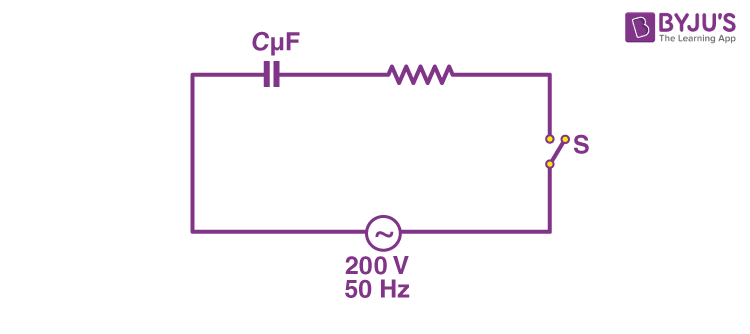P = V2/2

⇒ 500 = 1002/R

⇒ R = 20 Ω

Now across resistance 500 = I × 100

⇒ I rms = 5 A

Vrms = 200 V,

Vrms/real = 100 V

cos φ = 100/200 = ½ ⇒ Φ = 600

tan Φ = XC/ R = 1/ωRC

√3 = 1/100π(20)C

C = 1/(20π√3 x 100)

= 10-4 F

= 100 μF

Question 5) Find q on 4 μf capacity at t = ∞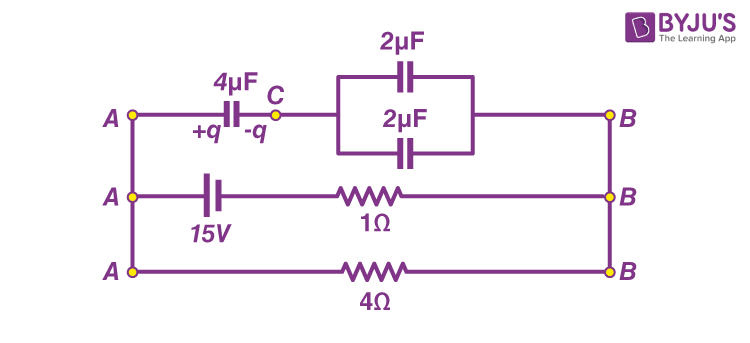(A) 8 μC

(B) 12 μC

(C) 20 μC

(D) 24 μC

Solution:

Using i = 15/(4+1) = 3 A

Therefore, VAB = i × 4 = 12 V

VAC = VCB = 6 Volt

Therefore, q on 4 μF = CVAC = 24 μC

Question 6) A parallel plate capacitor whose capacitance C is 14pF is charged by a battery to a potential difference V = 12 V between its plates. The charging battery is now disconnected and a porcelain plate with k = 7 is inserted between the plates, then the plate would oscillate back and forth between the plates, with a constant mechanical energy of ____ pJ.(Assume no friction)

Solution:

Initial energy stored in capacitor is,

Ui =(½) cv2

= (½) × 14 × (12)2 pJ

= 1008 pJ

Final energy stored in capacitor is,

Uf = Q2/2kC

$$\begin{array}{l}= \frac{(14\times 12)^{2}}{2\times 7\times 14}\end{array}$$

= 144 pJ

oscillating energy = Ui – Uf

= 1008 – 144

= 864 pJ

Question 7) A 2μF capacitor C1 is first charged to a potential difference of 10V using a battery. Then the battery is removed and the capacitor is connected to an uncharged capacitor C2 of 8 μF. The charge in C2 on equilibrium condition is ________ μC. (Round off to the Nearest Integer)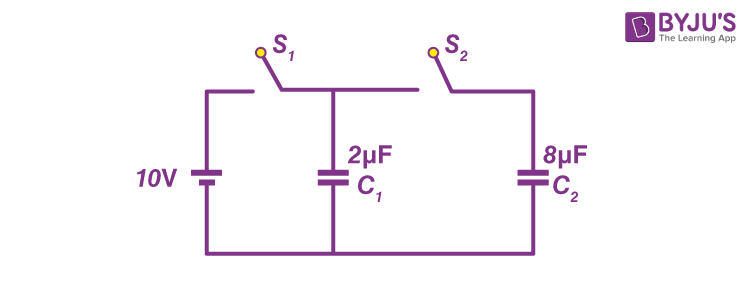Solution:

After capacitor C1 is fully charged,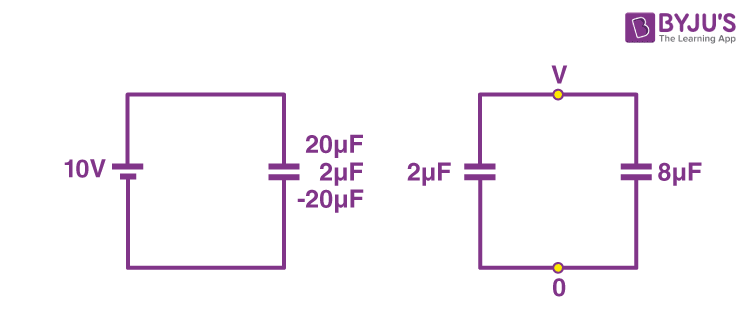When battery is removed & the capacitor is connected

At equilibrium condition, let voltage across each capacitor be V.

Then, using conservation of charge

2V + 8V = 20

10V = 20

V = 2 volt

Q = CV

Q = 8×2 = 16μc

Question 8) A parallel plate capacitor whose capacitance C is 14pF is charged by a battery to a potential difference V = 12 V between its plates. The charging battery is now disconnected and a porcelain plate with k = 7 is inserted between the plates, then the plate would oscillate back and forth between the plates, with a constant mechanical energy of ____ pJ.(Assume no friction)

Solution:

Initial energy stored in capacitor is,

Ui =(½) cv2

= (½) × 14 × (12)2 pJ

= 1008 pJ

Final energy stored in capacitor is,

Uf = Q2/2kC

$$\begin{array}{l}= \frac{(14\times 12)^{2}}{2\times 7\times 14}\end{array}$$

= 144 pJ

oscillating energy = Ui – Uf

= 1008 – 144

= 864 pJ

Question 9) A 2μF capacitor is charged as shown in figure. The percentage of its stored energy dissipated after the switch S is turned to position 2 is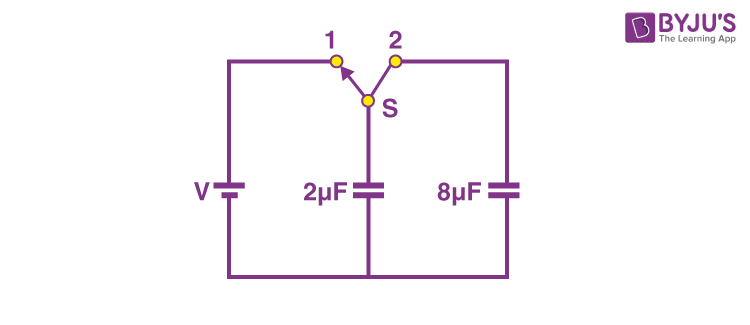(A) 0%

(B) 20%

(C) 75%

(D) 80%

Solution:

Initially, the energy stored in a 2μF capacitor is

Ui = (½)CV2 = (½) ( 2 x 10-6) V2 = V2 x 10-6 J

Initially, the charge stored in a 2 μF capacitor is Qi = Cv = (2 x 10-6) v = 2v x 10-6 coulomb. When switch S is turned to position 2,the charge flows and both the capacitors share charges till a common potential Vc is reached.

Vc = total charge/total capacitance

= (2v x 10-6)/( 2 + 8) x 10-6

= V/5 volt

Finally, the energy stored in both the capacitors

$$\begin{array}{l}U_{f}=\frac{1}{2}[(2+8)\times 10^{-6}](\frac{V}{5})^{2}=\frac{V^{2}}{5}\times 10^{-6}J\end{array}$$

Percentage loss of energy, ΔU = [(Ui – Uf)/Ui] x 100 %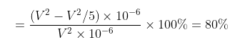= 80%

Question 10) In the given circuit, the charge on 4 μF capacitor will be

(A) 5.4 μF

(B) 9.6 μF

(C) 13.4 μF

(D) 24 μF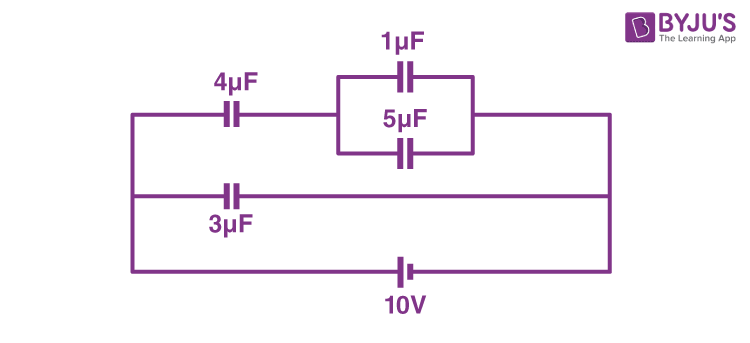Solution:

V1 + V2 = 10 ——(1)

4V1 = 6V2 ———-(2)

On solving equation (1) and (2) we get

V1 + (4V1/6) = 10

V1 + (2V1/3) = 10

3V1 + 2V1 = 30

5V1 = 30

⇒ V1 = 6 V

Charge on 4μf,

q = CV1 = 4 x 6 = 24 μF

Capacitor JEE Main Previous Year Questions With Solutions

### Parallel Plate Capacitor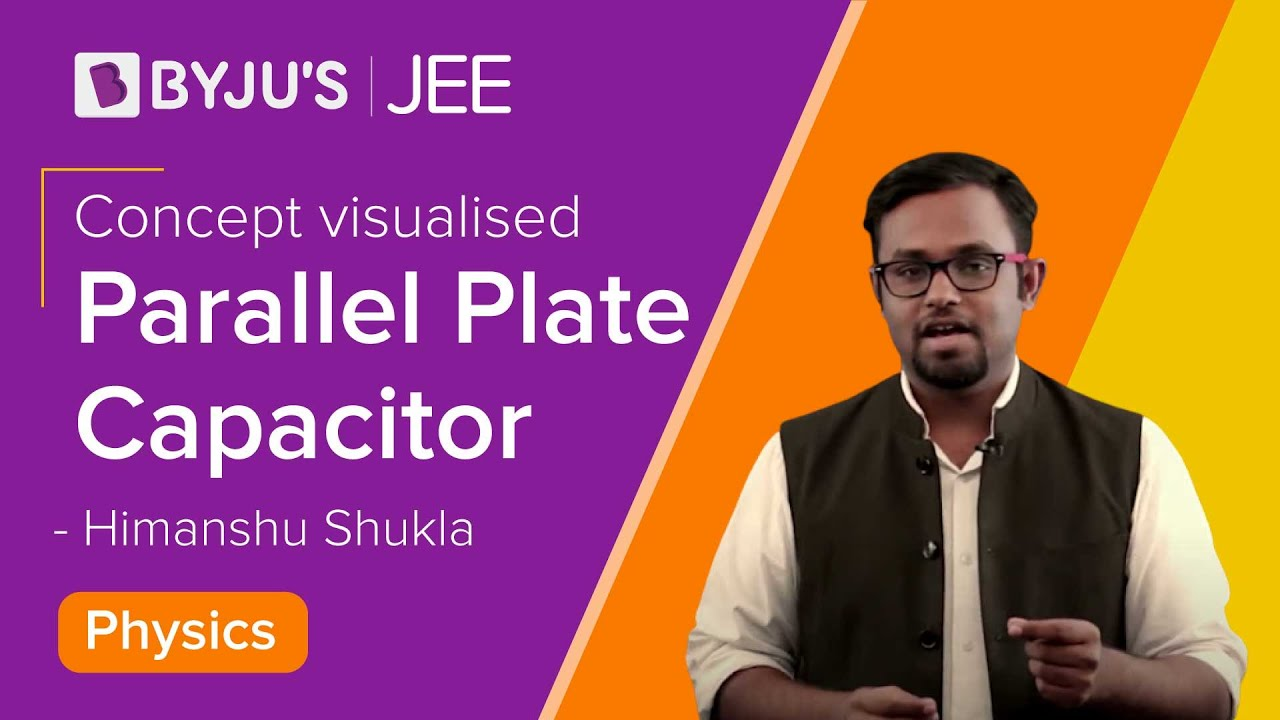### Capacitor Charging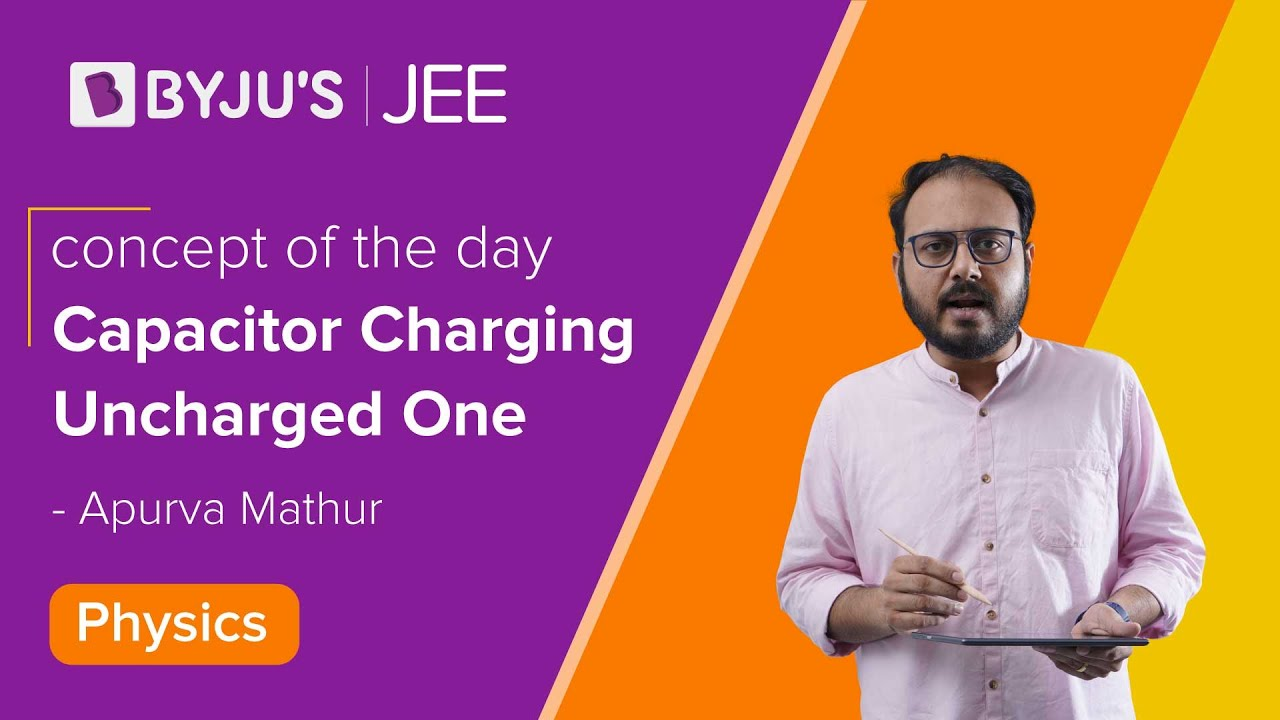### Top 10 Important Questions of Electrostatic Potential and Capacitance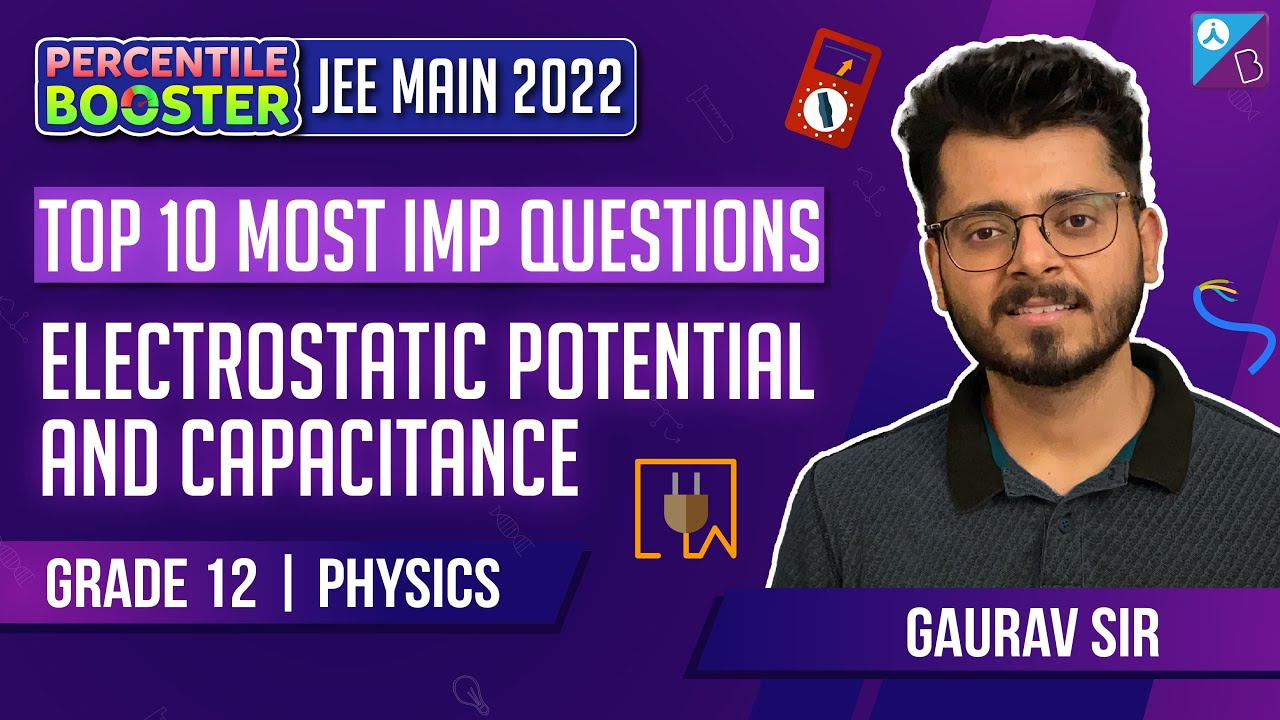### Capacitors and Electric Current – JEE Advanced PYQs